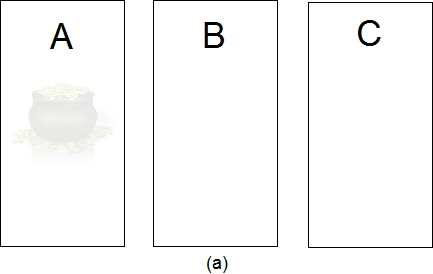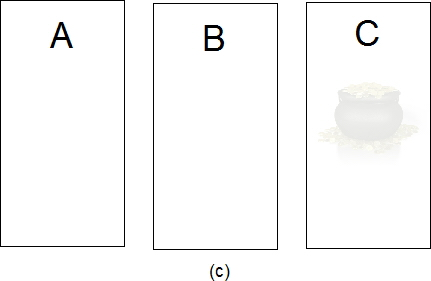## A Simple Solution to the Monty Hall Problem and Its Generalizations

### Introduction

The Monty Hall Problem is a simple brain-teaser that has stumped some very bright minds. It arose on the game show "Let's Make a Deal." A contestant is told that a prize lies behind one of three doors. After the contestant selects a door, the host, who knows where the prize resides, opens one of the two remaining doors showing that there is no prize there and asks if the contestant would like to change his or her mind and select the other remaining, unrevealed door. The question is: What should the contestant do?
Many people believe that it makes no difference whether the contestant switches. Given that there are now two doors, one with a prize and one without, many individuals (including for a while the great mathematician Paul Erdös) think that each door has a 50-50 chance of having the prize. For more information about the Monty Hall problem, its history and its solution, see the Wikipedia article on it.
The best course of action is for the contestant to switch. By doing so, the contestant doubles his or her chances of winning from 1/3 to 2/3. The easiest way to see this is that the contest originally had 1/3 of a chance of being correct and the opening of the door by the host has not changed this. Since the probability of the selected door having the prize plus the probability of the remaining door having the prize must sum to one, the latter must necessarily be 2/3. Below, we demonstrate this result through the enumeration of cases.
Of course, some people may think that the revealing of an empty door has altered the probability that the originally selected door is correct. Perhaps the easiest way to see that the two doors do not have the same probability of having the prize is to consider the following variation. Suppose there are 1000 doors, the contestant picks one and the host opens 998 other doors, all of whom have no prize. Originally, the contestant had one chance in a thousand of being correct, and this probability actually does not change after the host opens doors. The two remaining doors do not each have a 50-50 chance of having the prize. Think about it: by opening 998 doors is not the host leading the contestant in part to the correct door among the 999 if the prize resides behind one of them? Surely all this information about the 999 doors suggests that the one remaining unselected door is very likely to have the prize. Indeed, is it not suspicious that the host suddenly skips over one of the 999 doors?

### A Generalization and Its Solution

In this Jupiter Scientific Report, we shall consider the following generalization of the Monty Hall Problem. Suppose there are d doors. Behind p of these doors are prizes. After the contestant selects a door, the host opens r doors revealing no prizes behind them. By switching his or her choice, how does the contestant's chance of winning change?
Because the host cannot open a door with a prize behind it, there is a constraint on the value of r. Of the d-1 doors that the contestant has not picked, there might be p prizes among them thereby leaving at least d-1-p doors without prizes. Therefore, the number r of doors the host can reveal must be less than or equal to d-1-p otherwise the host might be forced to open a door with a prize behind it. In summary,

r ≤ d-1-p

In the simple example above, d=3, p=1, and r must be less than or equal to d-1-p=3-1-1=1 and, in fact, is 1.
Given that there are d doors, p of which have prizes behind them, the chance that a contestant picks a door with a prize is

pi = initial probability of winning = p/d            Eq.(1)

Now if one sums the probabilities of winning for all d doors, then one gets d × (p/d) = p. This sum must remain unchanged if the host reveals r doors without prizes. The originally selected door of the contestant has a chance of p/d of winning, while the d-1-r remaining doors must all have the same probability ps. Hence,

pi + (d-1-r) ps = p

Solving for ps, one finds

ps = p/d × (d-1)/(d-1-r)            Eq.(2)

Hence, if the contestant switches to one of the other doors, he or she will have a chance of ps of winning, which is larger than the originally chance of pi (= p/d) by

enhancement factor = (d-1)/(d-1-r)            Eq.(3)

For the single-prize (p=1), three-door (d=3), one-door-revealed (r=1) case, pi and ps are, according to the above formulas, respectively 1/3 and 2/3, which are the correct probabilities. The enhancement factor in this case is 2, meaning that is very much worth it to switch doors. If r = 0 so that no door is revealed, then the enhancement factor according to Eq.(3) is 1, meaning, as expected, that it makes no difference whether the contestant switches or not in this case.
As an example of equation (3), consider 10 doors, 2 prizes and 6 empty doors revealed by the host. The original chance of the contestant winning is 2/10 = 1/5. This is increased by the enhancement factor (10-1)/(10-1-6) = 3. So by switching, the contestant triples his or her chances to 3/5.

### Solution by Enumeriation

As an alternative derivation for the simplest case (p=1, d=3, r=1), one can enumerate the possibilities. Initially, there are three cases since the prize (a pot of gold) can be behind door A, B, or C:Without loss of generality, we can assume that the contestant selects door A since by symmetry the other two possibilities are equivalent. Each of the three cases in figure (a)-(c) is equally probable. If the contestant sticks with his or her choice, then the contestant wins a pot of gold in figure (a) and loses in figures (b) and (c), and hence the chance of winning is one out of three or 1/3. If the contestant switches, then he or she loses in figure (a) and but wins in figures (b) and (c) because after the host has revealed the door without the gold, the contestant will automatically pick the one with the gold. By switching the chances of winning are two out of three or 2/3.
For the mathematically inclined, one can do the enumeration-of-cases analysis for the general problem. There are two situations: (i) a prize is behind the initially selected door and (ii) a prize is not behind that door. In switching, one needs to compute the contributions from these two situations. For (i), there are Cd-1p-1 cases (which is the number of ways of placing p-1 prizes among the d-1 initially unselected doors) each of which has a probability of (p-1)/(d-1-r) of winning since there are p-1 prizes distributed among d-1-r doors. Here, Cnk is the number of different ways of placing k identical objects in n spaces:

Cnk = n!/(k! (n-k)!)

where n! means n factorial. For (ii), there are Cd-1p cases (which is the number of ways of placing p prizes among the d-1 unselected doors) each of which has a probability of p/(d-1-r) of winning. In total, there are Cdp cases, which is the number of ways of placing p prizes behind d doors, (and one can check that all cases are accounted for: Cd-1p-1 + Cd-1p = Cdp). Combining the results for the two situations leads to

ps = (Cd-1p-1 × (p-1)/(d-1-r) + Cd-1p × p/(d-1-r))/Cdp

which gives the same result as in Eq.(2) after some algebra is performed. Clearly, the method used above is simpler than the enumeration-of-cases approach.

This report was prepared by the staff of Jupiter Scientific, an organization devoted to the promotion of science through books, the internet and other means of communication.

This web page may NOT be copied onto other web sites, but other sites may link to this page.